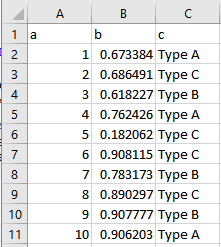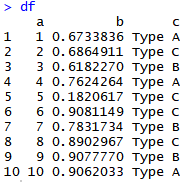# Lecture 5: Working with Data Part 1

## Working with Data

• R is all about working with data!
• Today we’re going to start going over the use of data.frames and tibbles
• data.frames are an object type; tibbles are basically data.frames with some extra bells and whistles, from the tidyverse package
• Most of the time, you’ll be doing calculations using them

## The Basic Idea

• Conceptually, data.frames are basically spreadsheets
• Technically, they’re a list of vectors## Example

• It’s a list of vectors… we can make one by listing some (same-length) vectors!
• (Note the use of = here, not <-)
``````df <- data.frame(RacePosition = 1:5,
WayTheySayHi = as.factor(c('Hi','Hello','Hey','Yo','Hi')),
NumberofKids = c(3,5,1,0,2))
df <- tibble(RacePosition = 1:5,
WayTheySayHi = as.factor(c('Hi','Hello','Hey','Yo','Hi')),
NumberofKids = c(3,5,1,0,2))
df``````
``````## # A tibble: 5 x 3
##   RacePosition WayTheySayHi NumberofKids
##          <int> <fct>               <dbl>
## 1            1 Hi                      3
## 2            2 Hello                   5
## 3            3 Hey                     1
## 4            4 Yo                      0
## 5            5 Hi                      2``````

## Looking Over Data

• Now that we have our data, how can we take a look at it?
• We can just name it in the Console and look at the whole thing, but that’s usually too much data
• We can look at the whole thing by clicking on it in Environment to open it up

## Glancing at Data

• What if we just want a quick overview, rather than looking at the whole spreadsheet?
• Down-arrow in the Environment tab
• `head()` (look at the head of the data - first six rows)
• `str()` (structure)
``str(df)``
``````## Classes 'tbl_df', 'tbl' and 'data.frame':    5 obs. of  3 variables:
##  \$ RacePosition: int  1 2 3 4 5
##  \$ WayTheySayHi: Factor w/ 4 levels "Hello","Hey",..: 3 1 2 4 3
##  \$ NumberofKids: num  3 5 1 0 2``````

## So What?

• What do we want to know about our data?
• What is this data OF? (won’t get that with `str()`)
• Data types
• The kinds of values it takes
• How many observations
• Variable names.
• Summary statistics and observation level (we’ll get to that later)

## Getting at Data

• Now we have a data frame, `df`. How do we use it?
• One way is that we can pull those vectors back out with `\$`! Note autocompletion of variable names.
• We can treat it just like the vectors we had before
``df\$NumberofKids``
``##  3 5 1 0 2``
``df\$NumberofKids``
``##  5``
``df\$NumberofKids >= 3``
``##   TRUE  TRUE FALSE FALSE FALSE``

## Quick Note

• There are actually many many ways to do this
• (some of which I even go over in the videos)
• For example, you can use `[row,column]` to get at data, for example `df\$NumberofKids >= 3` is equivalent to `df[,3] >= 3` or `df[,'NumberofKids']>=3`

## That Said!

• We can run the same calculations on these vectors as we were doing before
``mean(df\$RacePosition)``
``##  3``
``df\$WayTheySayHi``
``````##  Yo
## Levels: Hello Hey Hi Yo``````
``sum(df\$NumberofKids <= 1)``
``##  2``

## Practice

• Create `df2 <- data.frame(a = 1:20, b = 0:19*2,` `c = sample(101:200,20,replace=TRUE))`
• What is the average of `c`?
• What is the sum of `a` times `b`?
• Did you get any values of `c` 103 or below? (make a logical)
• What is on the 8th row of `b`?
• How many rows have `b` above 10 AND `c` below 150?

``````mean(df2\$c)
sum(df2\$a*df2\$b)
sum(df2\$c <= 103) > 0
df2\$b
sum(df2\$b > 10 & df2\$c < 150)``````
``##  152.7``
``##  5320``
``##  FALSE``
``##  14``
``##  6``

## The Importance of Rows

• So far we’ve basically just taken data frames and pulled the vectors (columns) back out
• So… why not just stick with the vectors?
• Because before long we’re not just going to be interested in the columns one at a time
• We’ll want to keep track of each row - each row is an observation. The same observation!

## The Importance of Rows

• Going back to `df`, that fourth row says that
• The person in the fourth position…
• Says hello by saying “Yo”
• And has no kids
• We’re going to want to keep that straight when we want to, say, look at the relationship between having kids and your position in the race.
• Or how the number of kids relates to how you say hello!
``````## # A tibble: 5 x 3
##   RacePosition WayTheySayHi NumberofKids
##          <int> <fct>               <dbl>
## 1            1 Hi                      3
## 2            2 Hello                   5
## 3            3 Hey                     1
## 4            4 Yo                      0
## 5            5 Hi                      2``````

## Working With Data Frames

• Not to mention, we can manipulate data frames and tibbles!
• Let’s figure out how we can:
• Create new variables
• Change variables
• Rename variables
• It’s very common that you’ll have to work with data a little before analyzing it

## Creating New Variables

• Easy! data.frames are just lists of vectors
• So create a vector and tell R where in that list to stick it!
• Use descriptive names so you know what the variable is
``````df\$State <- c('Alaska','California','California','Maine','Florida')
df``````
``````## # A tibble: 5 x 4
##   RacePosition WayTheySayHi NumberofKids State
##          <int> <fct>               <dbl> <chr>
## 1            1 Hi                      3 Alaska
## 2            2 Hello                   5 California
## 3            3 Hey                     1 California
## 4            4 Yo                      0 Maine
## 5            5 Hi                      2 Florida``````

## Our Approach - DPLYR and Tidyverse

• That’s the base-R way to do it, anyway
• We’re going to be using dplyr (think pliers) for data manipulation instead
• dplyr syntax is inspired by SQL - so learning dplyr will give you a leg up if you want to learn SQL later. Plus it’s just better.

## Packages

• tidyverse isn’t a part of base R. It’s in a package, so we’ll need to install it
• We can install packages using `install.packages('nameofpackage')`
``install.packages('tidyverse')``
• We can then check whether it’s installed in the Packages tab

## Packages

• Before we can use it we must then use the `library()` command to open it up
• We’ll need to run `library()` for it again every time we open up R if we want to use the package
``library(tidyverse)``
• There are literally thousands of useful packages for R, and we’re going to be using a few! Tidyverse will just be our first of many
• Google R package X to look for packages that do X.

## Varable creation with dplyr

• The mutate command will “mutate” our data frame to have a new column in it. We can then overwrite it.
• The pipe `%>%` says “take df and send it to that mutate command to use”
• Or we can stick the data frame itself in the `mutate` command
``````library(tidyverse)
df <- df %>%

## Creating New Variables

• We can use all the tricks we already know about creating vectors
• We can create multiple new variables in one mutate command
``````df <- df %>% mutate(MoreThanTwoKids = NumberofKids > 2,
One = 1,
KidsPlusPosition = NumberofKids + RacePosition)
df``````
``````## # A tibble: 5 x 7
##   RacePosition WayTheySayHi NumberofKids State MoreThanTwoKids   One
##          <int> <fct>               <dbl> <chr> <lgl>           <dbl>
## 1            1 Hi                      3 Alas~ TRUE                1
## 2            2 Hello                   5 Cali~ TRUE                1
## 3            3 Hey                     1 Cali~ FALSE               1
## 4            4 Yo                      0 Maine FALSE               1
## 5            5 Hi                      2 Flor~ FALSE               1
## # ... with 1 more variable: KidsPlusPosition <dbl>``````

## Manipulating Variables

• We can’t really change variables, but we sure can overwrite them!
• We can drop variables with `-` in the dplyr `select` command
• Note we chain multiple dplyr commands with `%>%`
``````df <- df %>%
select(-KidsPlusPosition,-WayTheySayHi,``````